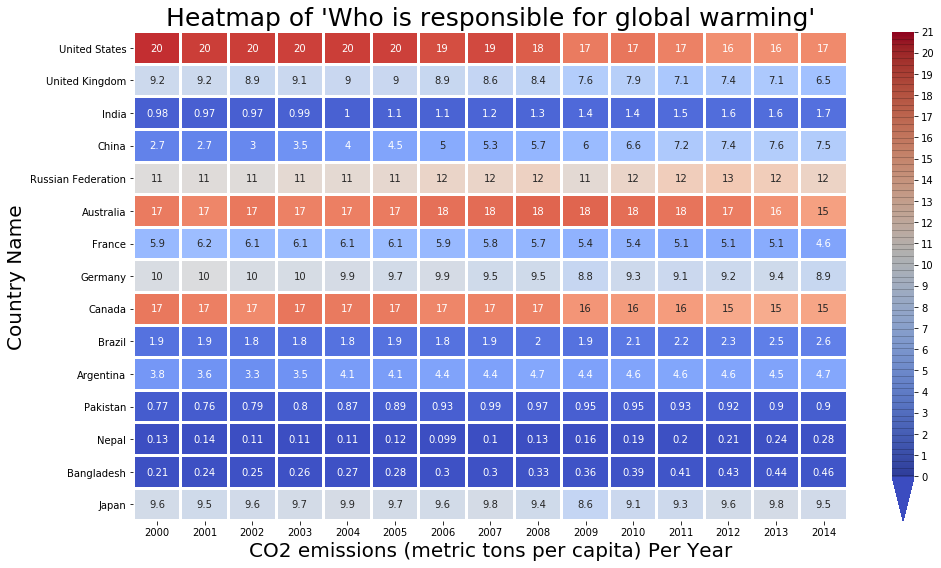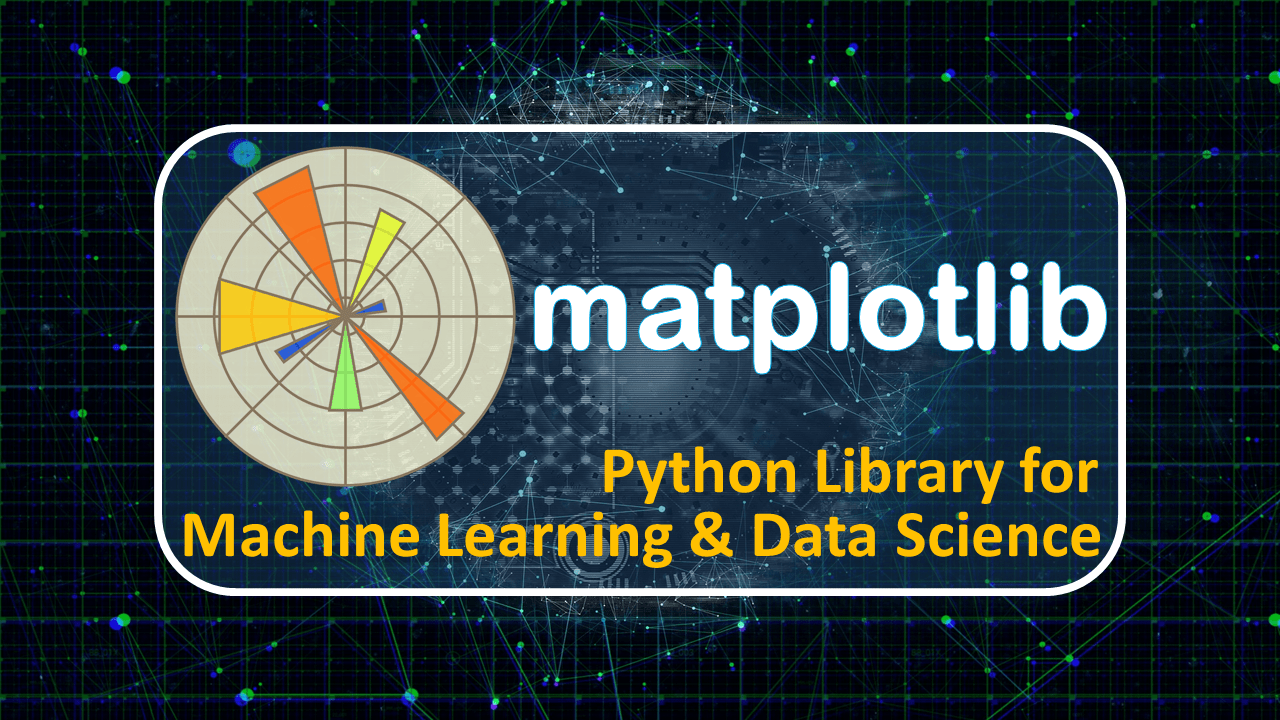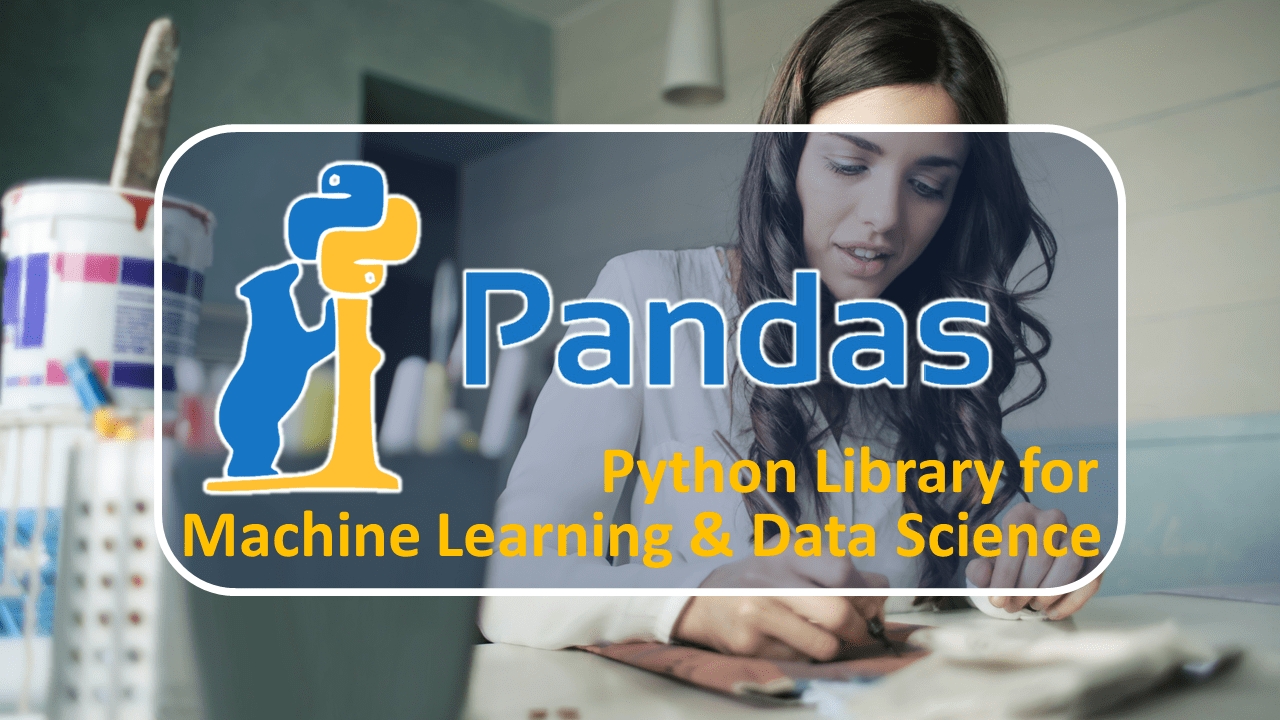## ML Project: Breast Cancer Detection Using Machine Learning Classifier

Breast cancer is a dangerous disease for women. If it does not identify in the early-stage then the result will be the death of the patient. It is a common cancer in women worldwide. Worldwide near about 12% of women affected by breast cancer and the number is still increasing. The doctors do not identify each and every breast cancer patient. That's the reason Machine Learning Engineer / Data...## ML Project: Directing Customers to Subscription Through Financial App Behavior Analysis

In this Machine Learning end to end project, we are working on financial application data and predict the customer who will take a premium version app subscription or not. Then the company will take action on the customers to give the offers or not. The data contain the customer's behavior and our job to find the insights from it. To complete this project, we use python programming and it's...## Seaborn Heatmap using sns.heatmap() | Python Seaborn Tutorial

In python seaborn tutorial, we are going to learn about seaborn heatmap or sns heatmap. The sns is short name use for seaborn python library. The heatmap especially uses to show 2D (two dimensional ) data in graphical format. Hey, don't worry. we will talk about step by step in later with practical. I hope, you are following python seaborn, matplotlib, numpy, and pandas tutorials because in...## Seaborn Scatter Plot using sns.scatterplot() | Python Seaborn Tutorial

If, you have x and y numeric or one of them a categorical dataset. You want to find the relationship between x and y to getting insights. Then the seaborn scatter plot function sns.scatterplot() will help. Along with sns.scatterplot() function, seaborn have multiple functions like sns.lmplot(), sns.relplot(), sns.pariplot(). But sns.scatterplot() is the best way to create sns scatter plot....## Seaborn Barplot – sns.barplot() 20 Parameters | Python Seaborn Tutorial

If you have x and y variable dataset and want to find a relationship between them using bar graph then seaborn barplot will help you. The seaborn sns.barplot() function draws barplot conveniently. In the seaborn histogram tutorial, we learned how to draw histogram using sns.distplot() function? But it doesn't support categorical dataset that's a reason, we are using sns barplot. Keep in mind sns...## Seaborn Histogram using sns.distplot() – Python Seaborn Tutorial

If you have numeric type dataset and want to visualize in histogram then the seaborn histogram will help you. For this seaborn distplot function responsible to plot it. In previous seaborn line plot blog learn, how to find a relationship between two dataset variables using sns.lineplot() function. Also, you are thinking about plot histogram using seaborn distplot because matplotlib plt.hist()...## Seaborn Line Plot – Draw Multiple Line Plot | Python Seaborn Tutorial

If you have two numeric variable datasets and worry about what relationship between them. Then Python seaborn line plot function will help to find it. Seaborn library provides sns.lineplot() function to draw a line graph of two numeric variables like x and y. Lest jump on practical. Import Libraries import seaborn as sns # for data visualization import pandas as pd # for data analysis import...## Python Seaborn Tutorial – Mastery in Seaborn Library

If you are working on data and try to find out insights from it. Then data visualization is the best way for that Python seaborn library is the best choice. Now, you are wondering about matplotlib because it also uses of data visualization. Don't worry, we will discuss it later. Why learn Seaborn & Who can? If you want to become a Machine learning engineer, Data Scientist, Data Analyst, etc....## Matplotlib imshow – Read & Show image using imread() & plt.imshow()

If you worry about, how to read and show an image using the matplotlib library then here you will get a solution for your problem. Along with that, you will be got a bonus. The matplotlib imshow() function helps to show the image. But plt.imshow() didn't work without mpimg.imread() function which is belongs to matplotlib.image module. So lets start practical. Import Libraries import...